# Pressure Force Area

Here we will learn about pressure, force and area, including what they are and how they are related to one another.

There are also pressure force area worksheets based on Edexcel, AQA and OCR exam questions, along with further guidance on where to go next if you’re still stuck.

## What are pressure, force and area?

Pressure, force and area are physical properties.

### What are pressure, force and area?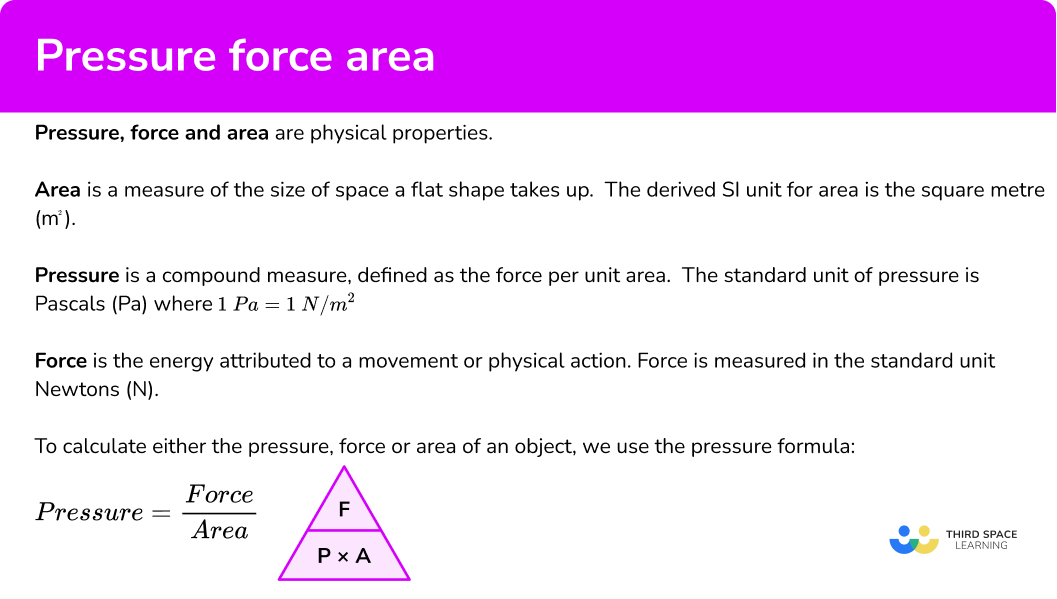### Area

Area is a measure of the size of space a flat shape takes up. The SI unit for area is the square metre (m^{2}) although we can use other units including square centimetres (cm^{2}), square millimetres (mm^{2}) or square inches (in^{2}) etc.

Area is inversely proportional to the pressure exerted; for a constant force, if the area in which the force is being applied to increases, the pressure exerted across that area decreases. We can therefore state that A\propto\frac{1}{P} for the area A and the pressure P. The constant of proportionality is the force, F.

Note: When we consider the area of an object, we are referring to the area of contact of the object with the floor / table / ground / wall / shelf etc.

### Force

Force is the energy attributed to a movement or physical action. Force is measured in the standard unit Newtons (N).

Newton’s Second Law of Motion states that force is equal to the mass of an object, multiplied by the acceleration, or F=MA. This is why weight and mass are not the same. Mass is a measure of how much matter there is in an object (usually measured in kilograms), whereas weight is the size of the pull of gravity on the object (measured in Newtons).

The acceleration due to gravity on the surface of the Earth is 9.807m/s^2 and so an average human with a mass of 62kg would have a weight of 62 \times 9.807=608N.

The force acting on an object is directly proportional to the pressure applied over a constant area. The greater the pressure, the greater the force for a constant area and vice versa. We can therefore state that F \propto P for the force F and the pressure P. The constant of proportionality is the area, A.

Step-by-step guide: Direct proportion

The forces used for this topic act on an object that is in contact with the floor / table / ground / wall / shelf etc. This means that the force will be perpendicular to the surface for all questions.

### Pressure

Pressure is a compound measure. Pressure is defined as the force per unit area. The standard unit of pressure is Pascals (Pa) where 1 \ Pa=1 \ N / {m^{2}}. We can also measure pressure using a Bar, where 1 Bar = 100,000 Pa = 100,000 \ N / {m^{2}}.

Imagine an elephant standing on 4 legs. The pressure applied to the ground is distributed over a wider area than if the elephant was standing on one leg. This is because the force remains constant (the weight of the elephant), but the area in which the force is applied has decreased, so the pressure increases. This is an example of inverse proportion.

As pressure is inversely proportional to the area, we can state that P\propto{1}{A} for the pressure P and the area A. The constant of proportionality is the force, F.

Step-by-step guide: Inverse proportion

To calculate either the pressure, force or area of an object, we use the pressure formula,

\text{Pressure}=\frac{\text{Force}}{\text{Area}}

Pressure triangle

This can be written as a formula triangle (sometimes called the pressure triangle).

We can circle what we are trying to find and the formula triangle tells how to calculate the unknown property.

## How to calculate pressure, force or area

In order to calculate pressure, force or area using the formula triangle:

1. Draw the triangle and circle the required property.
2. Substitute values for the other two properties and complete the calculation.
3. Write down the solution, including the units.

### Explain how to calculate pressure, force or area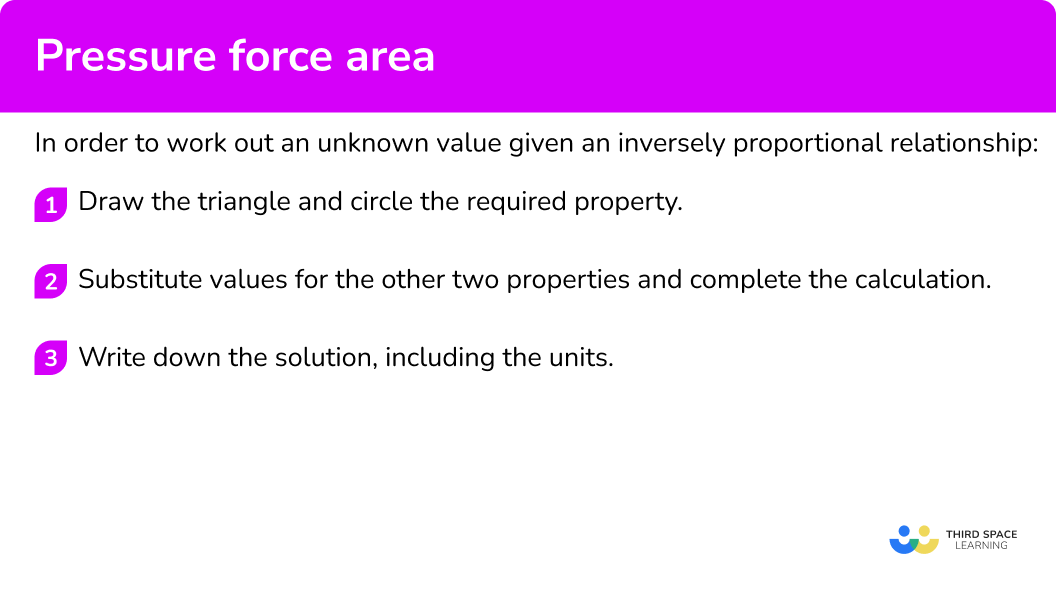### Related lessons oncompound measures

Pressure force area is part of our series of lessons to support revision on compound measures. You may find it helpful to start with the main compound measures lesson for a summary of what to expect, or use the step by step guides below for further detail on individual topics. Other lessons in this series include:

## Pressure force area examples

### Example 1: calculating pressure (Pa)

A force of 800 \ N acts on an area of 200 \ m^{2}. Calculate the pressure in Pascals.

1. Draw the triangle and circle the required property.

To calculate the pressure we would need to divide the force by the area.

2Substitute values for the other two properties and complete the calculation.

Substituting F=800N and A=200m^{2}, we have

800\div{200}=4

3Write down the solution, including the units.

Pressure = 4 \ N / {m^{2}}=4 \ Pa.

### Example 2: calculating pressure (Bar)

A force of 6.5 \times 10^6 \ N acts on an area of 0.2 \ m^{2}. Calculate the pressure in Bar.

To calculate the pressure we would need to divide the force by the area.

Converting 6.5 \times 10^6 to an ordinary number, we get F=6500000N .

P=6500000\div{0.2}=32500000

We currently have 32500000 \ Pa. As we want the solution in Bar, we need to divide the value by 100,000,

32500000\div{100000}=325 \ Bar.

### Example 3: calculating area (m2)

A force of 200 \ N exerts a pressure of 40 \ N/m^{2}. Calculate the area of the surface that the force is being applied to in square metres.

To calculate the area we would need to divide the force by the pressure.

Substituting F=200N and P=40 \ N / {m^{2}}, we have

A=200\div{40}=5

Area =5m^{2}.

### Example 4: calculating area (converting units of pressure)

A force of 950 \ N exerts a pressure of 53 \ Pa. Calculate the area in square metres, correct to 2 decimal places.

To calculate the area we would need to divide the force by the pressure.

As we need the units for the area to be in square metres, we need to convert the pressure from Pascals into N / {m}^{2}. As 1Pa=1N / {m}^{2}, we can simply state that our pressure P=53N / {m}^{2}.

Substituting F=950N and P=53N / {m}^{2} into the pressure triangle, we have

A=950\div{53}=17.9245283…

Area =17.92m^{2}\text{ (2dp)}.

### Example 5: calculating force (N)

A box has a cross sectional area of 1.2 \ m^2 and exerts a pressure of 60 \ N / {m}^{2} on the ground. Calculate the force that the box exerts on the ground, in Newtons.

To calculate the force we would need to multiply the pressure by the area.

As P=60 \ N / {m}^{2} and A=1.2 \ m^2, substituting these into the formula triangle we get,

F=60\times{1.2}=72

Force =72 \ N.

### Example 6: calculating force (converting units)

A pressure of 35 \ N/m^2 is exerted on an area of 40 \ 000 cm^{2}. Calculate the force in Newtons.

To calculate the force we would need to multiply the pressure by the area.

The area is in square centimetres, so we need to divide by 100^2 to find the area in square metres.

40 \ 000 \div{100}^{2}=4

Substituting P=35 \ N / {m^{2}} and A=4m^2 into the pressure triangle, we have

F=35\times{4}=140

Force = 140 \ N.

### Example 7: calculating pressure given the mass

An object has a mass of 2.4kg and makes contact with the surface of the Earth with an area of 14.9m^{2}. Given that the acceleration due to gravity g=9.807m/s^{2}, calculate the pressure exerted on the Earth by the object to 2 decimal places. State the units in your answer.

Before we use the formula triangle, we need to calculate the force exerted on the Earth by the object. This can be found by multiplying the mass by the acceleration due to gravity (F=MA).

F=2.4\times{9.807}=23.5368 \ N

As we want to determine the pressure, we need to divide the force by the area.

Now we have F=23.5268 \ N and A=14.9m^{2}.

Substituting these values into the pressure triangle, we have

P=23.5368\div{14.9}=1.579651007…

Pressure =1.58 \ N / {m^{2}}\text{ (2dp)}.

### Common misconceptions

• The area is the total surface area of the object

The area is the amount of surface in which the object is in contact with. This will usually be one face of an object, or given as the cross sectional area.

• Mixing up the placement of the letters in the triangle

Formula triangles have limited use and if the letters are incorrectly written in the wrong place, the subsequent calculation will be incorrect. To overcome this, the standard units of pressure are N/m^2 which is a force (N), divided by an area (m^{2}) and so P=F \div A.

### Practice pressure force area questions

1. A force of 800 \ N acts on an area of 20 \ m^{2}. Calculate the pressure.

0.4 \ N / {m^{2}}40 \ N / {m^{2}}4 \ N / {m^{2}}400 \ N / {m^{2}}Using the pressure triangle,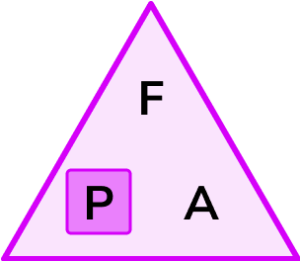and so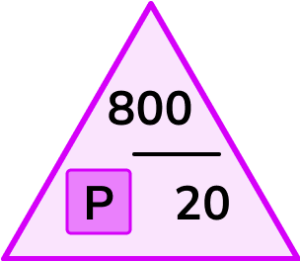P=800\div{20}=40 \ N / {m^{2}}

2. A force of 970 \ N acts on an area of 350 \ m^{2}. Calculate the pressure. Give your answer in Pascals correct to 3 significant figures.

2.78 \ Pa0.361 \ Pa0.36 \ Pa2.77 \ Paand so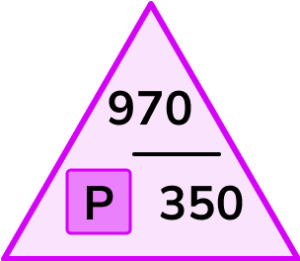P=970\div{350}=2.771428571 \ N / {m^{2}} = 2.77 \ Pa \text{ (3sf)}

3. A cabinet has a mass m=61.1kg and exerts a pressure of 380 \ N/m^2 on the surface of the Earth. Given that w=mg where g=9.807m/s^2 and w is the weight of an object in Newtons, calculate the area of the base of the cabinet correct to 2 decimal places.

0.63m^20.16m^21.58m^2227698.93m^2w=mg=61.1 \times 9.807=599.2077 \ N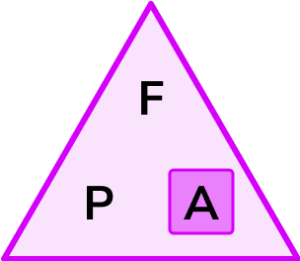and so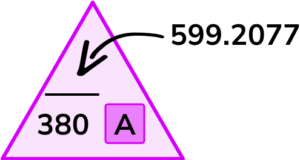\begin{aligned} &A=599.2077\div{380}=1.576862368…\\\\ &A=1.58m^{2}\text{ (2dp)} \end{aligned}

4. A force of 240 \ N exerts a pressure of 7.3 \ Pa. Calculate the area of the surface that the force is being applied to.

1752.0m^20.03m^230.4m^232.9m^21Pa=1N / {m}^{2}\text{ and so }7.3Pa=7.3N / {m}^{2}

Using the pressure triangle, we haveand so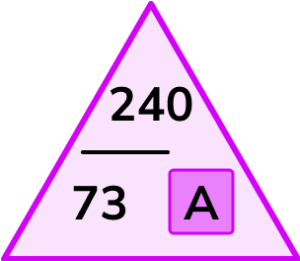\begin{aligned} &A=240\div{7.3}=32.87671233…\\\\ &A=32.9m^{2}\text{ (1dp)} \end{aligned}

5. A pressure of 40 \ N/m^2 is exerted on an area of 7 \ m^{2}. Calculate the force.

350 \ N280 \ N320 \ N380 \ N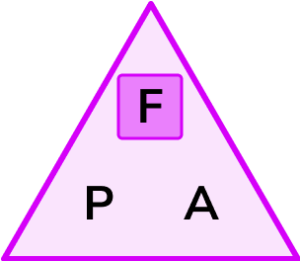and so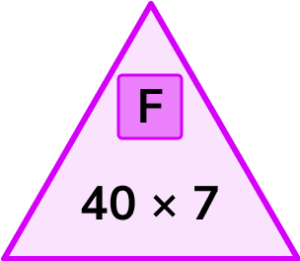F=40\times{7}=280 \ N

6. A pressure of 74 \ N/m^{2} is exerted on an area of 32000 \ cm^{2}. Calculate the force. Give the answer correct to 3 significant figures.

236 \ N23.1 \ N23.2 \ N237 \ NA = 32000cm^2 = 3.2m^2and so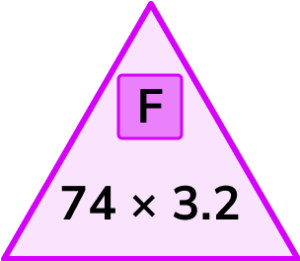F=74\times{3.2}=236.8 \ N

### Pressure force area GCSE questions

1. Work out the force when the pressure is 48 \ N/m^{2} and the area is 6 \ m^{2}. Circle your answer.

(1 mark)

288 \ N

(1)

2. Work out the area when the pressure is 35 \ N/m^2 and the force is 2100 \ N.

(2 marks)

2100\div 35

(1)

60 \ m^2

(1)

3. A block is resting on the floor.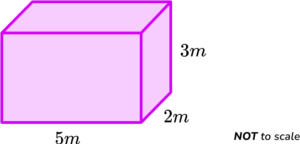The downwards force of the block is 7600 \ N.

Calculate the pressure exerted on the floor.

(3 marks)

\text{Area}=5\times 2=10

(1)

7600\div 10

(1)

760 \ N / {m^{2}}

(1)

## Did you know?

• Air pressure (atmospheric pressure) can change depending on where we are in the world. If we are up a mountain the air pressure is less than when we are at sea level.
• Each time a particle comes into contact with a wall, it exerts a force on the wall. If you increase the number of particles within the container (such as an inflating balloon), the frequency of collisions increases, and so does the pressure of the gas inside. At a high pressure, the balloon may not be able to withstand the increased amount of force on its surface, and so it bursts.

## Learning checklist

You have now learned how to:

• Convert between related compound units (pressure) in numerical and algebraic contexts

## Still stuck?

Prepare your KS4 students for maths GCSEs success with Third Space Learning. Weekly online one to one GCSE maths revision lessons delivered by expert maths tutors.

Find out more about our GCSE maths tuition programme.